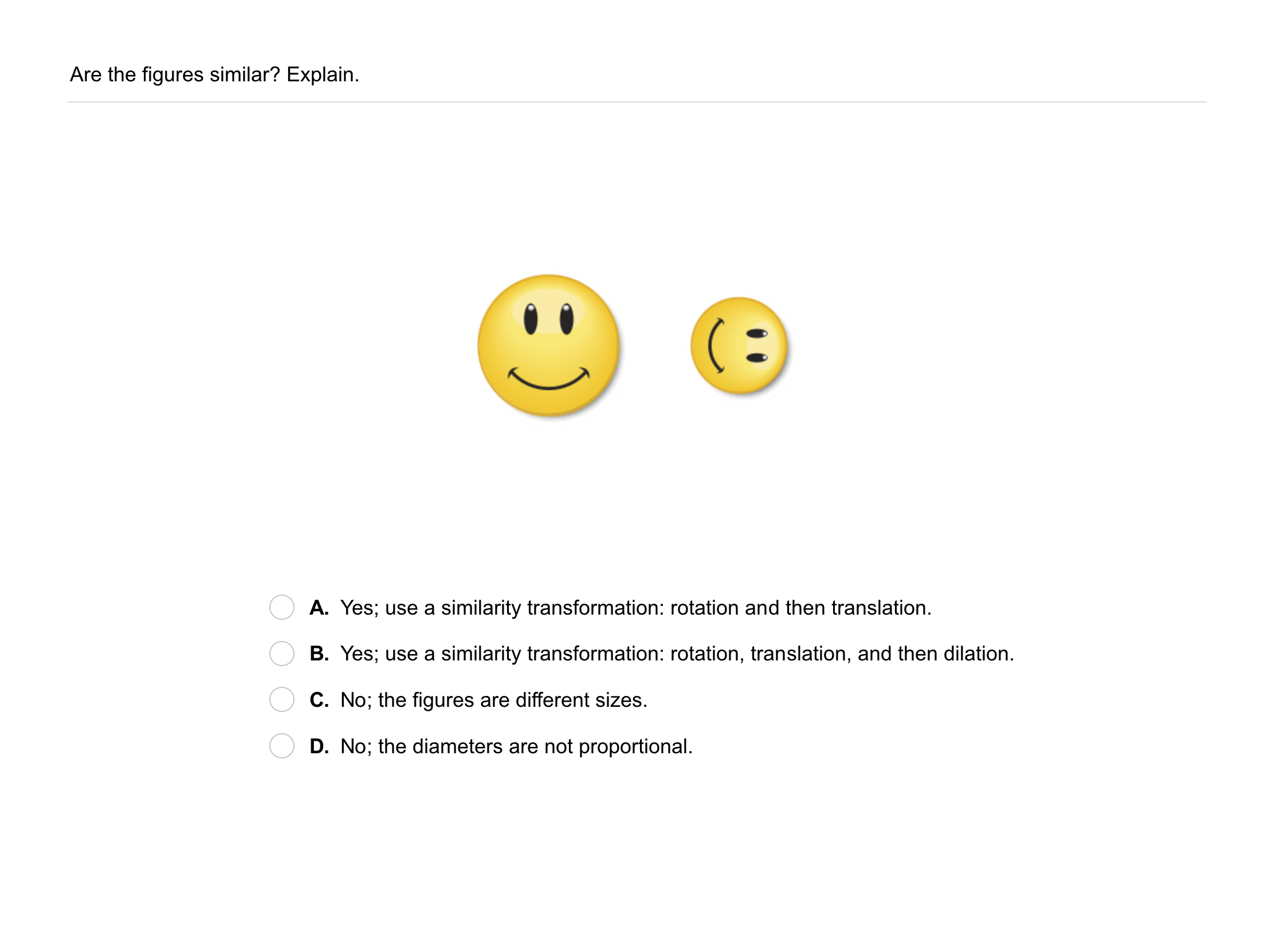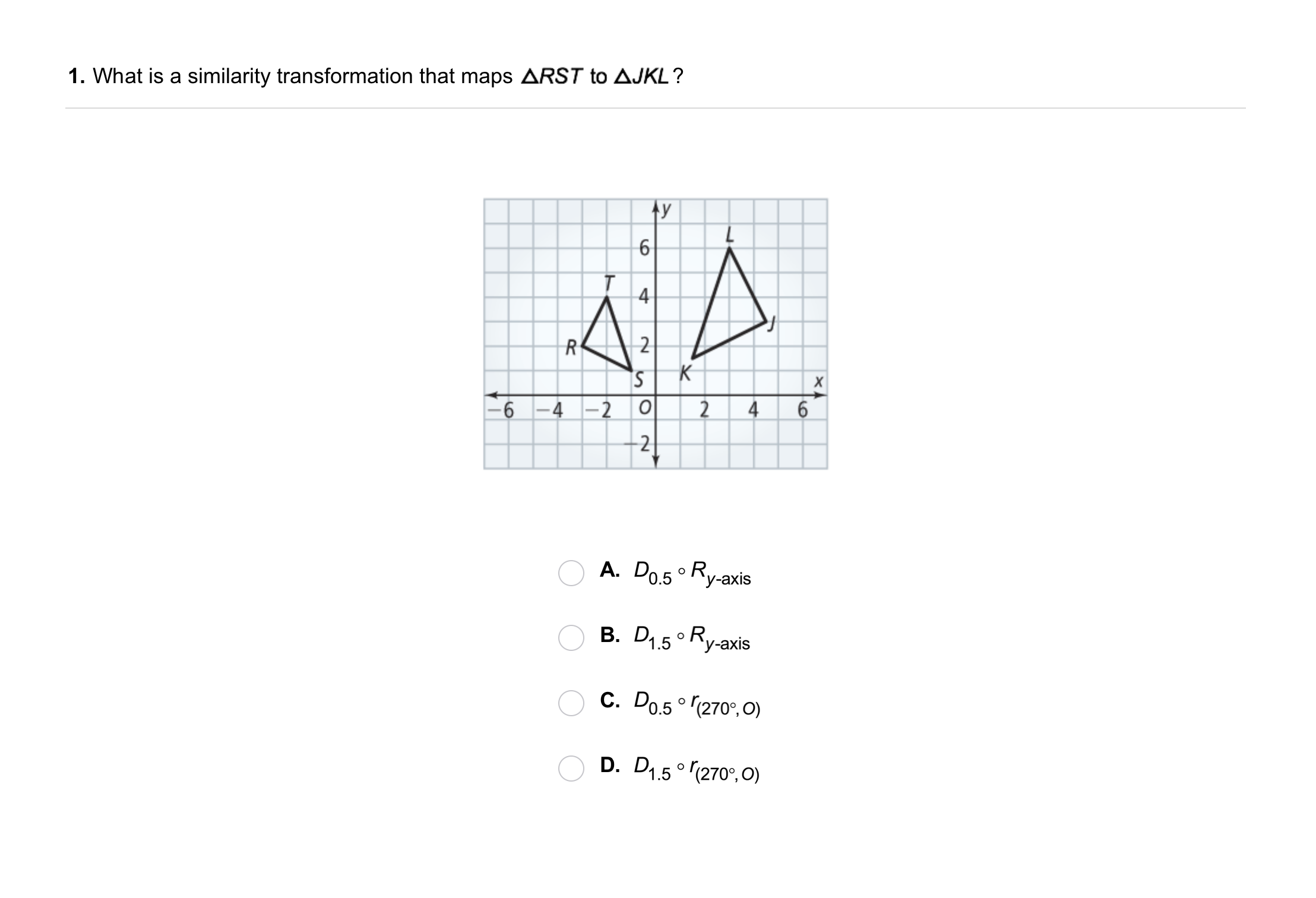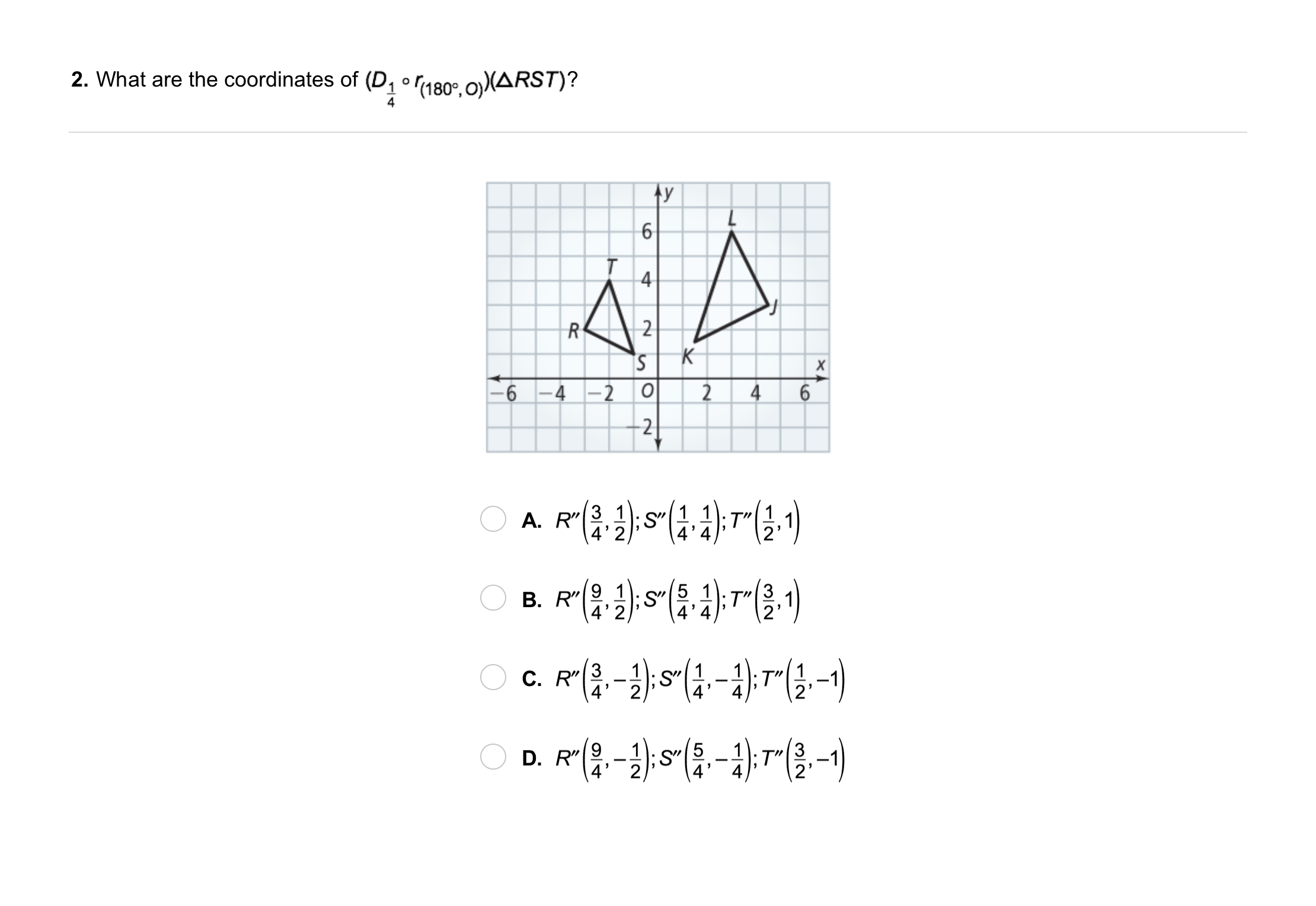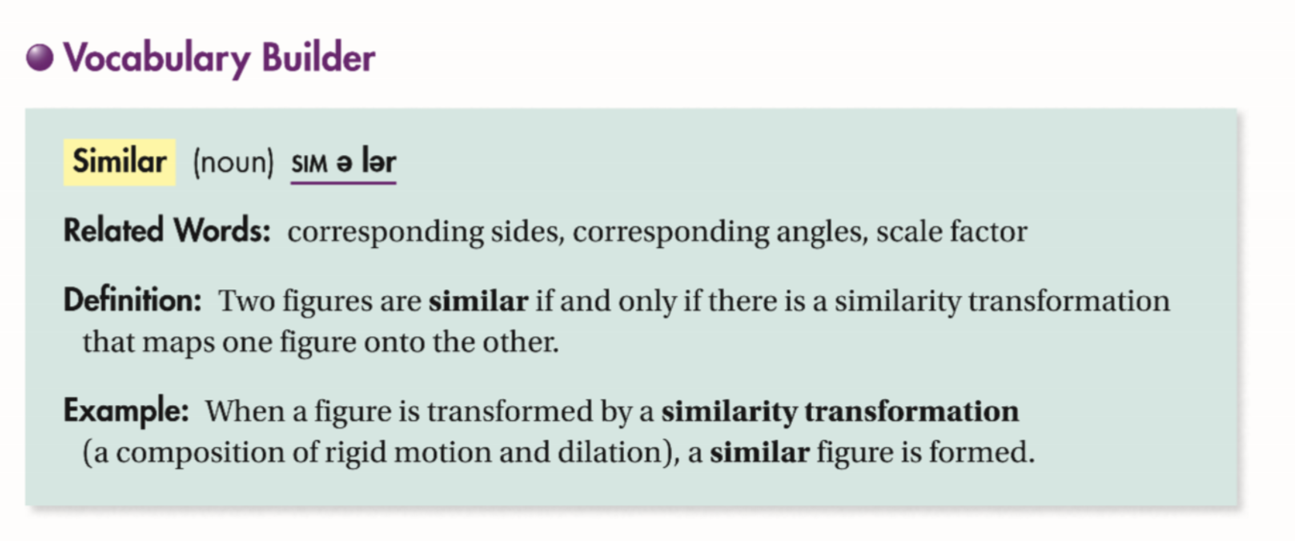Geometry 9-7 Similarity Transformations
starstarstarstarstarstarstarstarstarstar
by Matthew Richardson
| 17 Questions
Note from the author:
A complete formative lesson with embedded slideshow, mini lecture screencasts, checks for understanding, practice items, mixed review, and reflection. I create these assignments to supplement each lesson of Pearson's Common Core Edition Algebra 1, Algebra 2, and Geometry courses. See also mathquest.net and twitter.com/mathquestEDU.
The outlined content above was added from outside of Formative.
The outlined content above was added from outside of Formative.1
1
10 pts
Solve It! Your friend says that she performed a composition of transformations to map △ABC to △A'B'C'. Describe the composition of transformations.
The outlined content above was added from outside of Formative.
2
10 pts
Problem 1 Got It? Reasoning:LMN has vertices L(-4, 2), M(-3, -3), and N(-1, 1). Suppose the triangle is translated 4 units right and 2 unit up and then dilated by a scale factor of 0.5 with center of dilation at the origin. Sketch the △LMN in black and the resulting image of the composition of transformations in blue. Include all relevant graph detail.
The outlined content above was added from outside of Formative.3
3
10 pts
Problem 2 Got It?
A
B
C
DThe outlined content above was added from outside of Formative.4
4
10 pts
Problem 3 Got It?
A
B
C
D
The outlined content above was added from outside of Formative.5
5
10 pts
Problem 4 Got It?
A
B
C
D6
6
10 pts
A
B
C
D7
7
10 pts
A
B
C
D9
9
10 pts
Open-Ended: For △TUV, give the vertices of a similar triangle after a similarity transformation that uses at least 1 rigid motion.10
10 pts
Review Lesson 9-3: Which capital letters of the alphabet are rotation images of themselves? Draw each letter and give an angle of rotation (<360°).
11
10 pts
Review Lesson 6-7: Three vertices of an isosceles trapezoid are (-2, 1), (1, 4), and (4, 4). Graph the given vertices in black and all possible fourth vertices in blue. Label the vertices with coordinates. Include all relevant graph detail.
12
10 pts
Review Lesson 1-8: Find the area of each figure. Not all areas will be used.

• A square with 5-cm sides
• A rectangle with base 4.1 cm and height 7 cm
• A 4.7 cm-by-2.5 cm rectangle13
10 pts
Vocabulary Review: Complete each sentence with the most appropraite word(s) from the list.
• angle measure
• dilations
• distance
• rigid motion
• Rigid motions and dilations both preserve __?__.
• __?__ preserve distance.
• __?__ do not preserve distance.15
10 pts
Use Your Vocabulary: The image of a dilation is 5 in. and the corresponding preimage length is 2 in. What is the scale factor of the dilation?

16
10 pts
Use Your Vocabulary: The scale factor of a dilation is 2/3. An image length is 4 m. What is the corresponding preimage length?
2 m
3 m
4 m
5 m
6 m
17
10 pts
Reflection: Math Success